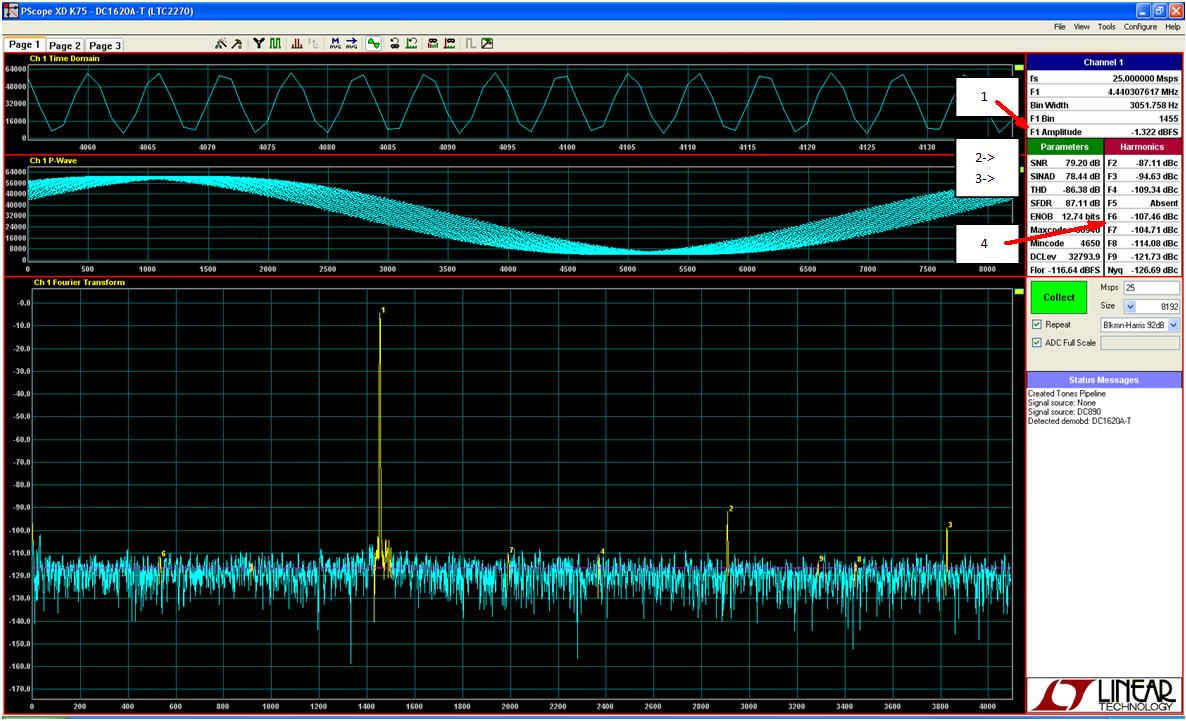# PScope Basics

### Introduction

When Linear Technology sends out a high speed ADC demo board we also provide the tools to evaluate that demo board. PScope is Linear Technology’s data collection software—it’s as simple as it is powerful. In order to get the demo board working you need to apply power to the demo board, a clock signal to the clock input, and an input signal. To get data sheet performance, filters will be required on the clock and analog inputs.   You will also need a data collection board. Linear Technology has provided several data collection boards; the DC718, DC890, and DC1371. The PScope software will accept data from all of them.

### Setup

When PScope is opened you will see three empty panels. Before the features of PScope can be used, data needs to be collected. PScope needs to be configured with the desired sample rate, transform size, and desired window. First enter the sampling frequency of the ADC, for example 25Msps (Point 1 in Figure 1 below). Then select the transform size and window you wish to use, in this case an 8k transform (Point 2 in Figure 1 below). If the sample clock and input signal are coherent signals then a window isn’t required. If they are non-coherent then the appropriate window should be selected. In this example the Blackman-Harris 92dB window is selected (Point 3 in Figure 1 below).Figure 1. PScope Setup

### Collecting Data

After the demo board is plugged in to the data collection board, power is applied and a clock signal is supplied, PScope automatically finds the connected demo board, and configures itself accordingly. Typically there is no reason to configure the demo board manually. The name of the demo board will appear in the status messages (Point 1 in Figure 2 below). In Figure 2 the DC890 is the data collection board, and the attached demo board is the DC1620A-T. After the collect button is hit the data will be displayed. The top window shows the input data, point for point, as it is sampled by the ADC. The X-axis shows the number of each sample as they are sampled by the ADC and the y-axis shows the value of the sample (Point 2 in Figure 2 below). The second window reconstructs one period of the waveform from the input data; this is called the PWave, or primitive wave (Point 3 in Figure 2 below). It only contains valid data when sampling coherently. When the data is sampled coherently it is a reconstruction of one period of the sampled waveform. The x-axis indicated where the sample is relative to the total number of samples, and the y-axis is the value of the sample. The third window is the FFT of the input data (Point 4 in Figure 2 below). The fundamental, and harmonics are labeled in yellow, and the blue line shows the average noise floor. The x-axis shows the bin number from 0 to fs/2 and the y-axis is the amplitude of each bin in dB relative to full scale of the ADC.Figure 2. PScope Collecting Data

### Results

The power of the fundamental can be monitored by looking at F1 amplitude (Point 1 in Figure 3 below). Typically all ADC testing is done 1dB below the full scale range of the ADC to avoid overdriving the ADC inputs. The SNR is 79.20dB shown here is relative to full scale, so another 1.32dB can be added in to give a total SNR of 80.52dB (Point 2 in Figure 3 below). Other important results are listed below SNR (Point 3 in Figure 3 below). SINAD, THD and ENOB are also calculated and displayed here. SFDR is calculated and shown as well. It is calculated by adding up the squares of the powers in the harmonics, and taking the square root. The DCLev shows what the DC offset is in terms of LSBs, and the noise floor shown is the average noise floor. In the right hand column we see the power in each of the harmonics relative to the amplitude of the carrier, or the fundamental (Point 4 in Figure 3 below). The noise floor is calculated by averaging all the FFT points in blue. It represents the SNR of the ADC, plus the processing gain of the transform, which is 10*log (Transform size/2).

This covers the basic setup of PScope. There are other advanced features of PScope that will be covered in later blog entries. PScope is a power tool that allows high speed ADCs to be evaluated quickly and accurately. This will allow you to spend more time on your design, and less time with the demo board.Figure 3. PScope Results